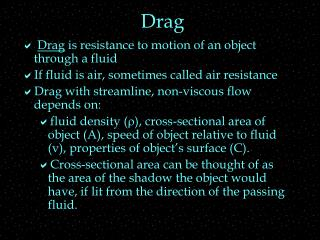Download PresentationDrag

Loading in 2 Seconds...

# Drag - PowerPoint PPT Presentation

Drag. Drag is resistance to motion of an object through a fluid If fluid is air, sometimes called air resistance Drag with streamline, non-viscous flow depends on:I am the owner, or an agent authorized to act on behalf of the owner, of the copyrighted work described.
Download Presentation## Drag

An Image/Link below is provided (as is) to download presentation

Download Policy: Content on the Website is provided to you AS IS for your information and personal use and may not be sold / licensed / shared on other websites without getting consent from its author.While downloading, if for some reason you are not able to download a presentation, the publisher may have deleted the file from their server.

- - - - - - - - - - - - - - - - - - - - - - - - - - E N D - - - - - - - - - - - - - - - - - - - - - - - - - -
Presentation Transcript
1. Drag • Drag is resistance to motion of an object through a fluid • If fluid is air, sometimes called air resistance • Drag with streamline, non-viscous flow depends on: • fluid density (r), cross-sectional area of object (A), speed of object relative to fluid (v), properties of object’s surface (C). • Cross-sectional area can be thought of as the area of the shadow the object would have, if lit from the direction of the passing fluid.

2. Drag • Depends on: • density of fluid (r), cross-sectional area of object presented to fluid (A), relative speed of object and fluid (v), properties of the object’s surface (C). • Direction: Always opposes relative motion of fluid and object • Note: This eqn doesn’t apply to viscous or turbulent flow

3. Projectiles and drag • An object moving vertically does not have the same vertical motion as an object that is moving sideways too, even if vyi is same, if drag is not negligible. • Drag force has a vertical component that depends on speed, not just vy

4. Relative Motion • Relativity means comparing quantities measured by one observer to • the same quantities measured • by another observer • in a different • Classical Relativity • is all we study in 201 • familiar • very good approximations at speeds much less than 300,000 km/s

5. Setup Demo • A, B, and C start out together (same x) • Relative to Earth • A goes 3 units each 10s • B goes 1 units each 10s • C stays at rest • Record the relative positions in the table below. Include signs!

6. t=0 t=0 t=0 t=10s t=10s t=10s t=20s t=20s t=20s t=30s t=30s t=30s t=0 t=0 t=10s t=10s t=20s t=20s t=30s t=30s Position DATA • Where is each person relative to you?

7. Relative Velocity • From the measured relative position as a function of time, • find the relative velocities in the table below: vAB= vBB= vCB= vAC= vBC=

8. Patterns • Check your relative velocity answers to see if they fit the expected patterns: • a) vBB=0  No one moves relative to themselves • Does this match your answers? • b) vCB = -vBC If you move East relative to the cows, the cows move West relative to you • Does this match your answers ? • c) vAC = vAB + vBC Vector addition of velocities • Does this match your answers?

9. Vector Addition of Velocities • A, B, and C each stand for an object, person, … • Each term is the velocity of • 1st subscript • relative to 2nd. • Pretend you are 2nd subscript and looking at 1st. • Beware of order of subscripts! • Beware of direction (or sign) of vector.

10. Example Red car is traveling at 60 mph in the positive direction relative to the road. Blue car is behind the red car traveling in the same direction at 65 mph. Find the velocity of the red car relative to the blue car. A= ______, B= ________, C= ________- vAC = vAC = vAB + vBC vAB = Why negative? vBC = vAB = ?

11. Example 2 A bicyclist rides 30 mph on a day with a 15 mph wind. When she rides into a headwind, she experiences a drag force of 100 N. What drag force does she feel going the opposite direction? What drag force does she feel after a 90-degree turn? A= _____, B= _______, C= ________ vAC = vBA = vBC = ?

12. Example 2 When she rides into a headwind: A= _____, B= _______, C= ________ vAC = vBA = vBC = ? When she rides with tailwind: A= _____, B= _______, C= ________ vAC = vBA = vBC = ? Since D is proportional to v2, we can do a ratio: D1/ v12 = D2/ v22 , so D2=

13. Example 2 A= bicyclist, B= air , C= earth vAC = 30 j vAB = ? vBC = 15 i vACx = vABx + vBCx 0 = vABx + 15 mph vABx = -15 mph vACy = vABy + vBCy 30mph = vABy + 0 vAB = 30 mph Since D is proportional to v2, we can do a ratio: D1/ v12 = D3/ v32 , so D3=